# 1. 列表视为矩阵

Python没有矩阵的内置类型。但是，可以将列表的列表视为矩阵。

``````A = [[1, 4, 5],
[-5, 8, 9]]``````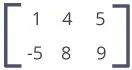# 2. 如何使用嵌套列表。

``````A = [[1, 4, 5, 12],
[-5, 8, 9, 0],
[-6, 7, 11, 19]]

print("A =", A)
print("A =", A)      # 第二行
print("A =", A)   # 第二行的第三元素
print("A[-1] =", A[-1])   # 第一行的最后一个元素
column = [];        # 空 list
for row in A:
column.append(row)

print("3rd column =", column)``````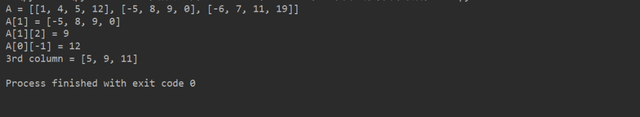# 1. 什么是NumPy？

NumPy是用于科学计算的软件包，它支持强大的N维数组对象。

# 2. 如何安装NumPy？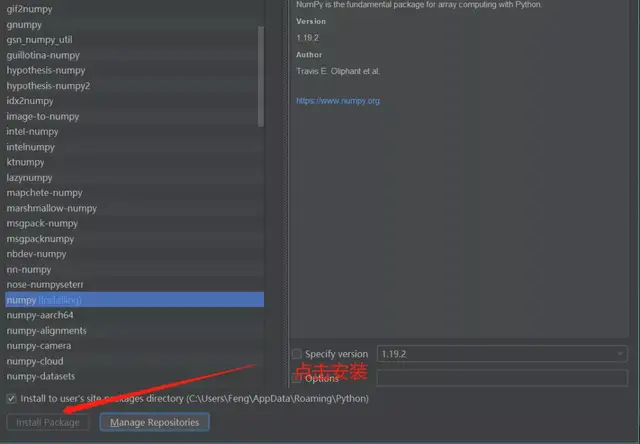NumPy提供数字的多维数组（实际上是一个对象）。

``````import numpy as np
a = np.array([1, 2, 3])
print(a)               # 输出: [1, 2, 3]
print(type(a))         # 输出: <class 'numpy.ndarray'>``````

NumPy的数组类称为ndarray。NumPy的数组类称为ndarray。

# 3.1 整数，浮点数和复数的数组

``````import numpy as np

A = np.array([[1, 2, 3], [3, 4, 5]])
print(A)

A = np.array([[1.1, 2, 3], [3, 4, 5]]) # 浮点数组
print(A)

A = np.array([[1, 2, 3], [3, 4, 5]], dtype = complex) # 复数数组
print(A)``````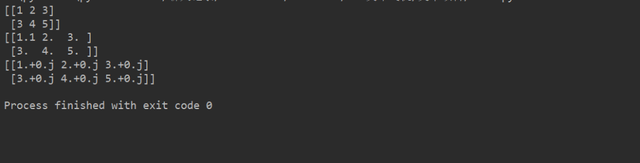# 3.2 零和一的数组

``````import numpy as np

zeors_array = np.zeros( (2, 3) )
print(zeors_array)
ones_array = np.ones( (1, 5), dtype=np.int32 ) // dtype
print(ones_array)      # 输出: [[1 1 1 1 1]]``````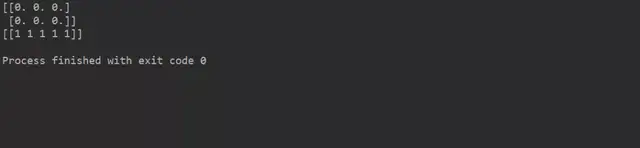3.使用arange()和shape()

``````import numpy as np

A = np.arange(4)

print('A =', A)

B = np.arange(12).reshape(2, 6)

print('B =', B)``````# 四、矩阵运算

``````import numpy as np

A = np.array([[2, 4], [5, -6]])
B = np.array([[9, -3], [3, 6]])
C = A + B      # 元素聪明的加法
print(C)``````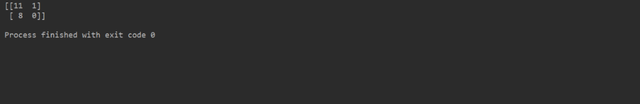``````import numpy as np

A = np.array([[3, 6, 7], [5, -3, 0]])
B = np.array([[1, 1], [2, 1], [3, -3]])
C = A.dot(B)
print(C)``````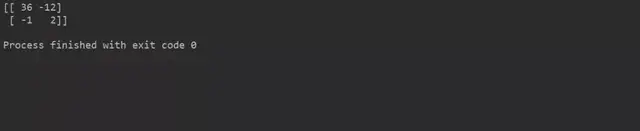``````import numpy as np

A = np.array([[1, 1], [2, 1], [3, -3]])
print(A.transpose())``````NumPy使的任务更加轻松。

# 1. 访问矩阵元素

``````import numpy as np
A = np.array([2, 4, 6, 8, 10])

print("A =", A)     # First element
print("A =", A)     # Third element
print("A[-1] =", A[-1])   # Last element``````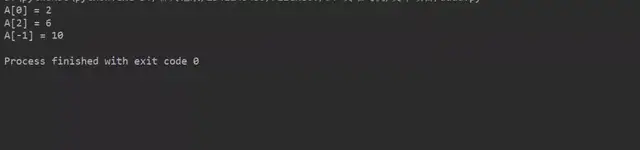``````import numpy as np

A = np.array([[1, 4, 5, 12],
[-5, 8, 9, 0],
[-6, 7, 11, 19]])

#  First element of first row
print("A =", A)

# Third element of second row
print("A =", A)

# Last element of last row
print("A[-1][-1] =", A[-1][-1])``````# 2. 访问矩阵的行

``````import numpy as np

A = np.array([[1, 4, 5, 12],
[-5, 8, 9, 0],
[-6, 7, 11, 19]])

print("A =", A) # First Row
print("A =", A) # Third Row
print("A[-1] =", A[-1]) # Last Row (3rd row in this case)``````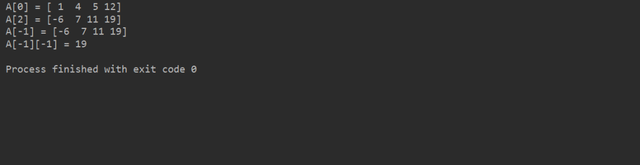# 3. 访问矩阵的列

``````import numpy as np

A = np.array([[1, 4, 5, 12],
[-5, 8, 9, 0],
[-6, 7, 11, 19]])

print("A[:,0] =",A[:,0]) # First Column
print("A[:,3] =", A[:,3]) # Fourth Column
print("A[:,-1] =", A[:,-1]) # Last Column (4th column in this case)``````